﻿ Confinement, Dark Matter and 11-dimensions Parallel UniversesInternational Journal of Theoretical and Mathematical Physics

p-ISSN: 2167-6844    e-ISSN: 2167-6852

2019;  9(5): 131-135

doi:10.5923/j.ijtmp.20190905.02### Confinement, Dark Matter and 11-dimensions Parallel Universes

Doron Kwiat

17/2 Erez Street, Mazkeret Batya, Israel

Correspondence to: Doron Kwiat, 17/2 Erez Street, Mazkeret Batya, Israel.
 Email: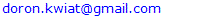Abstract

An 11-dimensions super universe is suggested here. It is made of (at least) two 4-dimensional universes. One of them is our own universe. A complete description of both universes requires 11 dimensions. 4 in each and additional 3 to describe their relative positions with respect to each other. Each universe is composed of Fermions and Bosons. The super universe has one field in common, namely the gravitation field which makes the fabric of it all. Curvatures in this fabric are the source for gravitational attraction forces between masses. Unlike gravitation, Fermions and Bosons are confined to their 4-dimensional universe. Based on momentum conservation, it is assumed that at the Big Bang instant, two universes were created simultaneously. Assuming two universes (ours and the parallel one) that are completely blind to each other except for gravity, allows, based on the confinement assumption, to suggest a model according to which the two parallel universes coexist, without one being aware of the other. Only gravitation can affect each other. This explains dark and gray matter as a consequence of overlapping universes. This work is an attempt to explain the connection between 4-dimensional elementary particles, gravitation, and 11-dimensions string theory particles, and the origin of dark matter.

Keywords: Fermions, Bosons, Confinement, Gravitation, Dark matter

Cite this paper: Doron Kwiat, Confinement, Dark Matter and 11-dimensions Parallel Universes, International Journal of Theoretical and Mathematical Physics, Vol. 9 No. 5, 2019, pp. 131-135. doi: 10.5923/j.ijtmp.20190905.02.

### 1. Introduction

If Big-Bang theory describes the moment of creation of our universe, there must have been at least two universes created simultaneously, because otherwise, momentum conservation is violated. This is of course true if the creation was due to an out-of-vacuum creation process (e.g. some primordial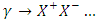A universe is made up of two kinds of particles. Massive ones (m>0), which must all obey Dirac's equation and are called fermions. And, massless ones (m=0) which are called Bosons.
Bosons move at a constant speed, the speed of light c. All massless particles, including photons, must move at same constant speed c.
The particles in the universe are attracted or repelled from each other due to forces exerted by massless bosons, which are the sources for all forces in any universe, except for gravitational forces.
Gravitation is a general force which affects all particles (massive and massless), both fermions and bosons. Gravitation has no particles to carry it. Rather, it is the result of curvatures in the fabric of spacetime.

### 2. Confinement

There is one unifying fact which applies to all kinds of particles in our universe. They are all confined in a 4-dimensional universe.
Fermions are confined in our 4-dimensional world as can be proven from Dirac equation structure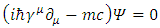(1)
and by use of group presentation theory and some manipulations with Dirac's matrices and their mathematics .
Bosons are confined to 4 dimensions as can be seen from the geodesic metric equation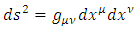(2)
which governs their motion and is based on the observational fact that c must be a universal constant. Adding more dimensions to the geodesic equation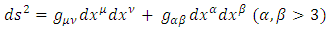(3)
will violate that observational fact (c will not be constant for all observers).
The only force that can affect particles irrespective of the universe in which they are confined, is gravitation. As mentioned, gravitation is a result of spacetime curvatures. These curvatures are not confined to our 4-dimensional universe. Rather they are present uninterruptedly in the super universe.
Now, as for the other universe. It was created in parallel to ours and has also 4 dimensions: 3 spatial coordinates and a single time coordinate. Its spatial and time coordinates may be different from our coordinates. It has particles like ours (probably similar), namely fermions and bosons.
The effect of the parallel universe on ours can only be mediated through gravitation.
We can never, and will never be able to, see or detect the presence of particles of the parallel universe since they are confined in their own 4-dimensional universe.
Because of this, our ability to feel that parallel universe is by its gravitational presence. This is the source for what we call "grey matter".
The other way to be able to tell that there is a parallel universe, is by looking at our mathematics. And indeed, to describe our universe in a complete manner, we need 4 dimensions for our universe. We need another three extra hidden dimensions to describe the location of that parallel universe with respect to ours. And, we need 4 additional dimensions for the parallel universe itself.
Altogether, if we ty to create one unifying theory of both universes together we will need 4+3+4 = 11 dimensions.

### 3. Types of Matter in a Universe

Suppose, all matter in our 4-dimensional universe is made up of massive (m>0) Fermions, and massless (m=0), force carrying Bosons.
Suppose now all, but gravitation, are confined to 4 dimensions.
This means that the other (parallel) universe cannot be detected in our universe, since its force carrying entities can never reach our universe (they are confined in their own universe).
The only effect we may be able to detect, is the effect of mass of that universe. Gravitation from that universe can reach out our universe.
This means, that when doing our gravitation calculations, there will be an extra matter present, which cannot be observed or explained as a result of solid matter.
We call this missing mass "Dark Matter". Dark matter [15-18] does not interact with neither bosons, nor with fermions. The existence of dark matter can only be inferred from the gravitational effect it seems to have on visible matter. Dark matter seems to outweigh visible matter roughly by a 6:1 ration.

### 4. Massless Fermions (Weyl) and the Neutrino

In the case of a massless fermion, m=0 and Dirac equation becomes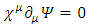(4)
This is Weyl's equation. It has a solution given by N=2 matrices [2,3]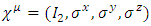(5)
where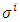are Pauli's 2x2 matrices, satisfying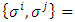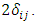One should not be misled here to take it as a 3-d representation, since the group will lose its Lorentz covariance. Also, it will lack the time component. Thus, this set should be written as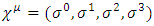(6)
with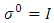and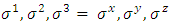It has 4 matrices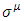satisfying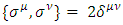and provides a a N=2 representation, in a d=4 universe, of a massless particle.
If neutrino has m=0, it cannot be a fermion since it will be a Weyl particle with d=4 N=2 which is impossible for a fermion under the requirement N2=2d. (N=2 requires d=2).
Otherwise, if m>0, it will fall under N=4, d=4 and can be a fermion of spin 1/2.
For an odd d (and an odd number of matrices), one should count the unit matrix I, as part of the group generators.
Therefore, a representation, based on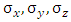is not a proper representation of fermions.

### 5. Photons are Confined to 4-dimensions

Photons dynamics are governed by 4-dimensions equation of motion: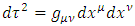(7)
With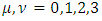and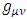the 4x4 gravitational 4-dimensions space metric.
If photons were to exist in more than 4 dimensions we would have: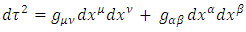(8)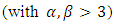and the speed of light c,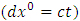will not be fixed, as should be, independent from reference frames.
Thus, photons must be confined in a 4-dimensional universe.
As a matter of fact, based on same metric principle, all massless particles (Bosons) must be confined in a 4-dimensional universe.

### 6. Fermions are Confined to 4-dimensions

Using group representation theory, it was recently shown, that Fermions must be confined to 4-dimensions .
The proof is based on Dirac equation (all Fermions are actually Dirac particles) and on the Dirac matrices (denoted here by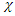the generalized matrices of n-dimensions instead of the conventional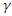) anticommutation restriction (known as Clifford's constraint ):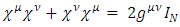(9)
This result was reached based on the following assumptions:
There exist, d Dirac gamma NxN matrices, where both d and N are integers.
These gammas are Lorentz covariant.
The gammas must obey the Clifford algebra canonical anti-commutation relation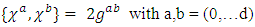(10)
The number d specifies the total number of world coordinates, of which there are the usual x,y,z spatial coordinate, at least one is a temporal coordinate and the rest are extended coordinates (may be temporal and may be spatial). By convention, in our 4 dimensional world we use d=3+1, namely x,y,z and t).
Based on the calculus of the Dirac gamma matrices and their traces, it can be proven, that the existence of an irreducible group G, made of NxN matrices of size 2d, is equivalent to the demand that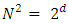(11)
Adding pairs of gamma matrices over d=4, results in reducible representations. Hence d>4 is impossible.
The conclusion is that all matter particles (Dirac fermions) are confined in a 4-dimensional universe.
As is obvious from the confinement proof, it relies on the Clifford condition and hence, it is the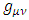term which connects the Fermions confinement theorem to gravity.

### 7. Gravitation is not Confined to 4-dimensions

According to the general theory of relativity, gravitational influences (attraction forces) are exerted by curvatures in the spacetime fabric. So far there is no evidence of any 4-dimensional confinement on gravitation. Space distortions can occur across universes in other dimensions.
As a matter of fact, gravitation and strings can be united if one assumes 11 dimensions [11-16].
This stands in contrast to Fermions and massless Bosons being confined to 4-dimensions.
It will therefore be assumed, that gravitation can pass freely between parallel universes.
One possible explanation of the need for 11 dimensions is the following:
4-dimensions are needed to describe an event in one universe. 3 dimensions are required to point to the position of the second universe with respect to the first. Another set of 4 dimensions is required to describe the position of an event in the second universe. Thus 4+3+4 = 11 dimensions are a must in a combined double universe.
So basically, one may assume that before the creation of the universes, there was an ultimate universe mad of gravitational field fabric. No forces of gravitation existed as there were no masses present. Once the two parallel universes emerged out of the ultimate universe, masses were created and dispersed in that gravitational fabric and their presence created curvatures in this gravitational field fabric. These curvatures created forces of attraction (which we call gravitation).

### 8. Parallel Universes and Higher Dimensions

In the quest for a unified theory, strings [3-14] are candidates for unified quantum theory of gravity. They demand a higher dimensional universe (11 -26).
For string theory to be consistent, space must have six or even seven space dimensions in addition to the three we know from everyday life.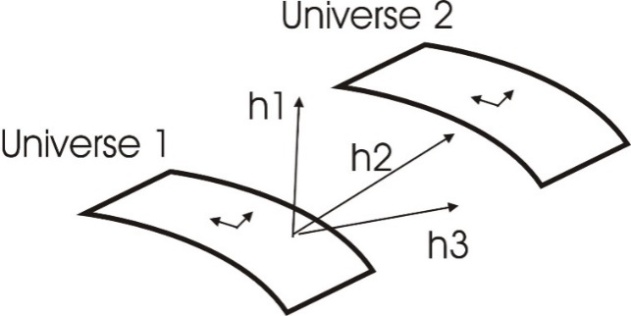Figure 1. A super universe made of two 4-dimensional universes. 3 hidden coordinates h1 h2 h3 are required to describe the relative positions of the two universes (branes) with respect to each other. Resulting in a total 11-dimensions super universe
There is no contradiction between the Fermionic and Bosonic confinement to 4-dimensions and the gravitation theories demanding 11 dimensions.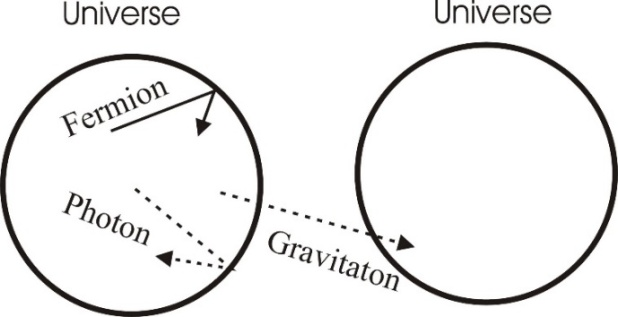Figure 2. Fermions and Bosons are confined in 4d. Gravitation is not confined to 4 dimensions

### 9. A Parallel Universe

From the beginning, there is a super universe made of a spacetime fabric (gravitation field). According to big bang theory, and based on momentum conservation, there must be at least two universes created simultaneously and moving in opposite directions (momentum must be conserved).
Suppose next, that in parallel to our universe, at the moment of creation, there were other universes created, parallel to ours. Those parallel universes may have the exact same content and size similar to our own universe, but with maybe a different particles/anti-particles ratio.
However, Fermions have a restriction – our material (fermionic) universe must be 4 dimensional.
A Fermion, cannot cross from one universe to a second universe because Fermions can only exist in 4 dimensions. This creates a confinement rule on Fermions. They are confined to that universe in which they exist (created in) and cannot cross to other universes.
Likewise, fermions from other universes can never cross into our material universe.
No massive particle in our universe can pass to another universe. However, photons and gravitation can pass freely between parallel universes.
Obviously, we will only have 4-dimensions in our fermionic universe, but there need to be more dimensions to include other parallel universes.
Since fermions can never exit their 4-dimensional universe, we say that fermions are confined.
The picture is thus the following:
We live in a 4-dimensional universe. Our universe is made of massive particles called Fermions. We can never exit our universe to other universes.
Suppose now, that Bosons, (e.g. photons), can pass freely from one universe to another. Thereof, information can be passed between these universes and so can forces of electromagnetism and gravitation.
But if a fermion exists in a parallel universe and confined in it, we will be able to observe it because light and gravitation can penetrate and transfer between the two parallel universes. This creates a contradiction because it means that fermions can exist in more than 4 dimensions.
The possible solution is the following.
All Bosons (including photons) are confined just as fermions to 4 dimensions. This means that the fermions in the parallel universe can never be detected by us, but they can affect us by their mass (through gravitation).
So, we will assume now that fermions and photons are confined to 4 dimensions while gravitation is free to transfer between the two parallel universes.
This will explain "dark matter". A parallel universe which affects ours via gravitation, but can never penetrate our universe and can never be observed by us. Only its mass affects our universe.
How can photons be confined to 4 dimensions?
If we look at Maxwell's equation, we can see its similarity to Dirac's equation. Same reasons for the confinement of Dirac's particles to 4 dimensions, can be applied to Maxwell's particles.
Here is a summary of what we have:
1. Fermions cannot exist in a universe of other than 4 dimensions.
2. There may exist at least two parallel universes, each of 4 dimensions.
3. Fermions may exist in each universe separately.
4. Fermions are confined inside a universe and cannot transfer between universes.
5. According to Maxwell's equation, and the constancy of the speeds of light, Photons can be considered to be confined in 4-dimensional.
6. Hence, Fermions and Photons are confined in 4-dimensional universes.
7. Gravitation is not confined.
Therefore, Fermions may be affected by gravitational forces from another universe, but cannot be seen outside their universe.
This may be the clue to "dark matter", where unexplained gravitational forces are predicted to exist, however no massive particles are observed, to justify these forces.

### 10. Dark Matter and Dark Energy

The visible universe  —including Earth, the sun, other stars, and galaxies—is made of Fermions and Bosons. Perhaps one of the most surprising discoveries of the 20th century was that this matter makes up less than 5 percent of the mass of the universe.
The rest of the universe appears to be made of a mysterious, invisible substance called dark matter  (25 percent) and a force that repels gravity known as dark energy  (70 percent).
One has not yet observed dark matter directly. It doesn't interact with any matter and it's completely invisible to light and other forms of electromagnetic radiation, making dark matter impossible to detect with current instruments. But, it exists because of the gravitational effects it appears to have on galaxies and galaxy clusters.
For instance, according to standard physics, stars at the edges of a spinning, spiral galaxy should travel much slower than those near the galactic center, where a galaxy's visible matter is concentrated. But observations show that stars orbit at more or less the same speed regardless of where they are in the galactic disk. This result makes sense if one assumes that the boundary stars are feeling the gravitational effects of an unseen mass.
Dark matter could also explain certain optical illusions that astronomers see in the deep universe. For example, pictures of galaxies that include strange rings and arcs of light could be explained if the light from even more distant galaxies is being distorted and magnified by massive, invisible clouds of dark matter in the foreground-a phenomenon known as gravitational lensing.
Dark energy was discovered in 1998 with by two international teams that included (Nobel Prize Laureates 2011) Adam Riess, Saul Perlmutter and Brian Schmidt. Previously, it was assumed that the attractive force of gravity would slow down the expansion of the universe over time. But when these two independent teams tried to measure the rate of deceleration, they found that the expansion was actually speeding up. We think that the accelerated expansion of the universe is driven by a kind of repulsive which seems to be growing stronger as the universe expands. We call this mysterious force dark energy.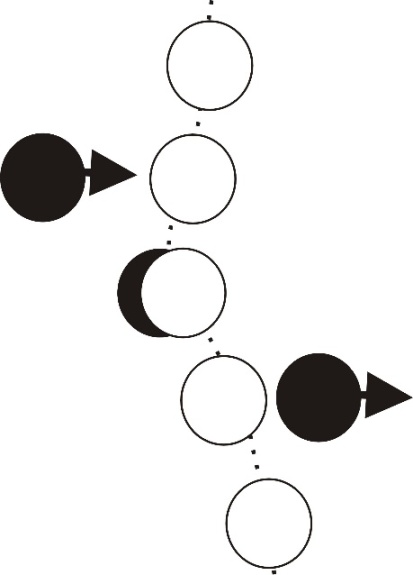Figure 3. A dark matter collision with regular matter will create a unique observable path diversion due to "unexplained" gravitational attraction
So what is dark matter and dark energy made of?
One possible explanation is that they are fermions and bosons from the parallel universe which is overlapping partially or fully, our universe. Parallel fermions are the dark matter while parallel bosons ae the dark energy.

### 11. Conclusions

Based on Fermionic and Bosonic confinement to a 4-dimensional universe, it is suggested, that only gravitational field from one universe can affect another universe. Even overlapping parts of universes can have mutual influences on one another. All other particles, whether massive (Fermions) or massless (Bosons) must be confined in their original 4-dimensional universe.
Therefore, based on the suggested theory, one may not be able to directly detect dark matter (which is part of a parallel universe). No interaction is possible between the two universes, (by any interactions, irrespective of their energies) except for gravitational attraction effects.

### References

  Dirac, P. A. M., "The Quantum Theory of the Electron" , Proceedings of the Royal Society A: Mathematical, Physical and Engineering Sciences. 117 (778): 610. 1928.  Kwiat D. "Fermions in d Dimensions". International Journal of Theoretical and Mathematical Physics 2019, 9(1): 14-19 DOI: 10.5923/j.ijtmp.20190901.03.  West P.C., Supergravity, Brane Dynamics and String Duality. Cambridge |University Press, 1999.  Y. Nambu, Strings, monopoles, and gauge fields, Phys. Rev. D 10, 4262. 1974.  M.B. Green, J.H. Schwarz, E. Witten “Superstring Theory” (Cambridge Monographs on Mathematical Physics, Cambridge University Press, 1987). In two volumes.  E Antonyan "Supergravities in Diverse Dimensions" June 10, 2003.  Craig Callender and Nick Huggett."Physics meets philosophy at the Planck scale. Contemporary theories in quantum gravity". paperback ISBN 0-511-04065-2 eBook. Cambridge University Press 2004.  Polchinsky J, "Superstring theory and beyond string theory". Vol II. Cambridge University Press, 2005.  Shiroman Prakasha and Ritam Sinhab, A complex fermionic tensor model in d dimensions. Journal of High Energy Physics, Volume 2018, Issue 2, article id.86, 25 pp.  Oscar Varela, Complete D = 11 embedding of SO(8) supergravity. Phys. Rev. D 97, 045010 (2018).  Brian Hatfield, Quantum Field Theory of Point Particles and Strings. Mathematical Physics Research, CRC Press, 2018, Taylor & Francis Group.  Grassi, A., Hatsuda, Y. and Mariño, M., Topological Strings from Quantum Mechanics, Ann. Henri Poincaré 2016 17: 3177. https://doi.org/10.1007/s00023-016-0479-4.  A.Polyakov, Fine structure of strings, Nuclear Physics B. Volume 268, Issue 2, 1986, pp 406-412.  J. Louis, T. Mohaupt, and S. Theisen, String Theory: An Overview, Max Planck Institute for theoretical Physics, http://www.aei.mpg.de/~theisen/LMT.pdf.  Freeman, K.C. (June 1970). "On the Disks of Spiral and S0 Galaxies". The Astrophysical Journal. 160: 811–830. Bibcode:1970ApJ...160..811F.  https://www.nationalgeographic.com/science/space/dark-matter.  Drees, M.; Gerbier, G. (2015). "Dark Matter" (PDF). Chin. Phys. C. 38: 090001.  P. J. E. Peebles, Bharat Ratra "The cosmological constant and dark energy". Review of Modern Physics, vol. 75, 2003.